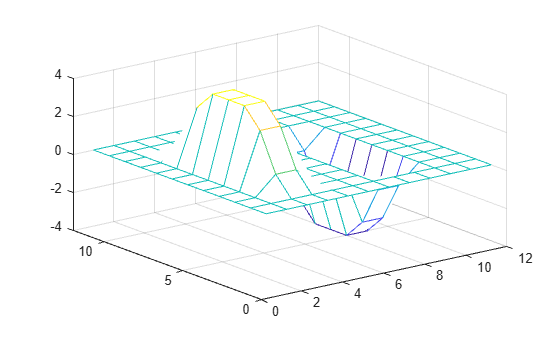# conv2

2-D convolution

## Syntax

``C = conv2(A,B)``
``C = conv2(u,v,A)``
``C = conv2(___,shape)``

## Description

example

````C = conv2(A,B)` returns the two-dimensional convolution of matrices `A` and `B`.```

example

````C = conv2(u,v,A)` first convolves each column of `A` with the vector `u`, and then it convolves each row of the result with the vector `v`.```

example

````C = conv2(___,shape)` returns a subsection of the convolution according to `shape`. For example, `C = conv2(A,B,'same')` returns the central part of the convolution, which is the same size as `A`.```

## Examples

collapse all

In applications such as image processing, it can be useful to compare the input of a convolution directly to the output. The `conv2` function allows you to control the size of the output.

Create a 3-by-3 random matrix `A` and a 4-by-4 random matrix `B`. Compute the full convolution of `A` and `B`, which is a 6-by-6 matrix.

```A = rand(3); B = rand(4); Cfull = conv2(A,B)```
```Cfull = 6×6 0.7861 1.2768 1.4581 1.0007 0.2876 0.0099 1.0024 1.8458 3.0844 2.5151 1.5196 0.2560 1.0561 1.9824 3.5790 3.9432 2.9708 0.7587 1.6790 2.0772 3.0052 3.7511 2.7593 1.5129 0.9902 1.1000 2.4492 1.6082 1.7976 1.2655 0.1215 0.1469 1.0409 0.5540 0.6941 0.6499 ```

Compute the central part of the convolution `Csame`, which is a submatrix of `Cfull` with the same size as `A`. `Csame` is equal to `Cfull(3:5,3:5)`.

`Csame = conv2(A,B,'same')`
```Csame = 3×3 3.5790 3.9432 2.9708 3.0052 3.7511 2.7593 2.4492 1.6082 1.7976 ```

The Sobel edge-finding operation uses a 2-D convolution to detect edges in images and other 2-D data.

Create and plot a 2-D pedestal with interior height equal to one.

```A = zeros(10); A(3:7,3:7) = ones(5); mesh(A)```Convolve the rows of `A` with the vector `u`, and then convolve the rows of the result with the vector `v`. The convolution extracts the horizontal edges of the pedestal.

```u = [1 0 -1]'; v = [1 2 1]; Ch = conv2(u,v,A); mesh(Ch)```To extract the vertical edges of the pedestal, reverse the order of convolution with `u` and `v`.

```Cv = conv2(v,u,A); mesh(Cv)```Compute and plot the combined edges of the pedestal.

```figure mesh(sqrt(Ch.^2 + Cv.^2))```## Input Arguments

collapse all

Input array, specified as a vector or matrix.

Data Types: `double` | `single` | `int8` | `int16` | `int32` | `int64` | `uint8` | `uint16` | `uint32` | `uint64` | `logical`
Complex Number Support: Yes

Second input array, specified as a vector or a matrix to convolve with `A`. The array `B` does not have to be the same size as `A`.

Data Types: `double` | `single` | `int8` | `int16` | `int32` | `int64` | `uint8` | `uint16` | `uint32` | `uint64` | `logical`
Complex Number Support: Yes

Input vector, specified as a row or column vector. `u` convolves with each column of `A`.

Data Types: `double` | `single` | `int8` | `int16` | `int32` | `int64` | `uint8` | `uint16` | `uint32` | `uint64` | `logical`
Complex Number Support: Yes

Second input vector, specified as a row or column vector. `v` convolves with each row of the convolution of `u` with the columns of `A`.

Data Types: `double` | `single` | `int8` | `int16` | `int32` | `int64` | `uint8` | `uint16` | `uint32` | `uint64` | `logical`
Complex Number Support: Yes

Subsection of the convolution, specified as one of these values:

• `'full'` — Return the full 2-D convolution.

• `'same'` — Return the central part of the convolution, which is the same size as `A`.

• `'valid'` — Return only parts of the convolution that are computed without zero-padded edges.

## Output Arguments

collapse all

2-D convolution, returned as a vector or matrix. When `A` and `B` are matrices, then the convolution `C = conv2(A,B)` has size `size(A)+size(B)-1`. When `[m,n] = size(A)`, ```p = length(u)```, and `q = length(v)`, then the convolution `C = conv2(u,v,A)` has `m+p-1` rows and `n+q-1` columns.

When one or more input arguments to `conv2` are of type `single`, then the output is of type `single`. Otherwise, `conv2` converts inputs to type `double` and returns type `double`.

Data Types: `double` | `single`

collapse all

### 2-D Convolution

For discrete, two-dimensional variables A and B, the following equation defines the convolution of A and B:

`$C\left(j,k\right)=\sum _{p}\sum _{q}A\left(p,q\right)B\left(j-p+1,k-q+1\right)$`

p and q run over all values that lead to legal subscripts of A(p,q) and B(j-p+1,k-q+1).

## Version History

Introduced before R2006a